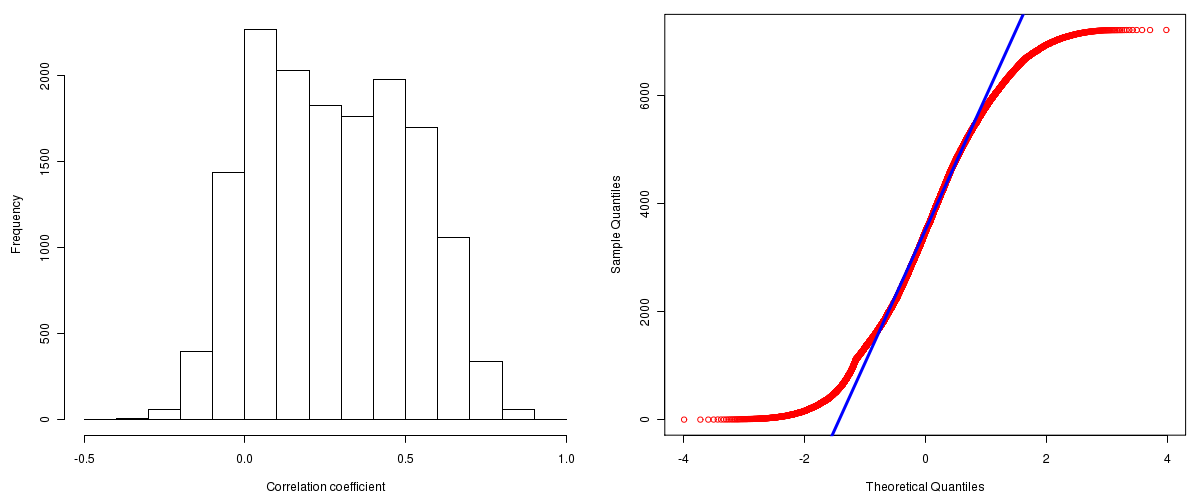Lung Squamous Cell Carcinoma: Correlations between copy number and mRNA expression
Maintained by John Zhang (Dana-Farber Cancer Institute)
Overview
Introduction

A TCGA sample is profiled to detect the copy number variations and expressions of genes. This pipeline attempts to correlate copy number and expression data of genes across samples to determine if the copy number variations also result in differential expressions. This report contains the calculated correlation coefficients based on measurements of genomic copy number (log2) values and intensity of the expressions of the corresponding feature across patients. High positive/low negative correlation coefficients indicate that genomic alterations result in differences in the expressions of mRNA the genomic regions transcribe.

Summary

The correlation coefficients in 10, 20, 30, 40, 50, 60, 70, 80, 90 percentiles are -0.0237, 0.04822, 0.114, 0.18764, 0.2695, 0.35196, 0.43392, 0.50798, 0.59764, respectively.

Results
Correlation results

Number of genes and samples used for the calculation are shown in Table 1. Figure 1 shows the distribution of calculated correlation coefficients and quantile-quantile plot of the calculated correlation coefficients against a normal distribution. Table 2 shows the top 20 features ordered by the value of correlation coefficients.

Table 1.  Counts of mRNA and number of samples in copy number and expression data sets and common to both

Category Copy number Expression Common
Sample 211 154 136
Genes 29390 17815 15551

Figure 1.  Summary figures. Left: histogram showing the distribution of the calculated correlations across samples for all Genes. Right: QQ plot of the calculated correlations across samples. The QQ plot is used to plot the quantiles of the calculated correlation coefficients against that derived from a normal distribution. Points deviating from the blue line indicate deviation from normality.Table 2.  Get Full Table Top 20 features (defined by the feature column) ranked by correlation coefficients

feature r p-value q-value chrom start end geneid
LSM1 0.9233 0 0 8 38140014 38153183 27257
ZNF639 0.8967 0 0 3 180524245 180536014 51193
ASH2L 0.8913 0 0 8 38082223 38116216 9070
DDHD2 0.8876 0 0 8 38208264 38238143 23259
ORAOV1 0.8785 0 0 11 69189512 69199346 220064
C9orf82 0.875 0 0 9 26830683 26882725 79886
WHSC1L1 0.8746 0 0 8 38251717 38358947 54904
PPFIA1 0.8734 0 0 11 69794471 69908150 8500
FADD 0.8678 0 0 11 69726917 69731144 8772
PSMD8 0.8634 0 0 19 43557061 43566304 5714
BAG4 0.8631 0 0 8 38153263 38187694 9530
PLAA 0.8621 0 0 9 26894518 26937138 9373
ACTL6A 0.862 0 0 3 180763402 180788889 86
DCUN1D1 0.8601 0 0 3 184143253 184181020 54165
RPL35A 0.8571 0 0 3 199161449 199167119 6165
TOPORS 0.8555 0 0 9 32530542 32542601 10210
POLR2H 0.8496 0 0 3 185563888 185569057 5437
ATP11B 0.8483 0 0 3 183993985 184122117 23200
PSMA1 0.8425 0 0 11 14482998 14621739 5682
SENP2 0.8421 0 0 3 186786725 186831583 59343
Methods & Data
Input

Gene level (TCGA Level III) expression data and copy number data of the corresponding loci derived by using the CNTools package of Bioconductor were used for the calculations. Pearson correlation coefficients were calculated for each pair of genes shared by the two data sets across all the samples that were common.

Correlation across sample

Pairwise correlations between the log2 copy numbers and expressions of each gene across samples were calculated using Pearson correlation.